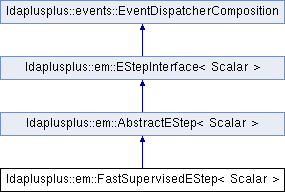LDA++
ldaplusplus::em::FastSupervisedEStep< Scalar > Class Template Reference

#include <FastSupervisedEStep.hpp>

Inheritance diagram for ldaplusplus::em::FastSupervisedEStep< Scalar >:## Public Types

enum  CWeightType { Constant = 1, ExponentialDecay }

## Public Member Functions

FastSupervisedEStep (size_t e_step_iterations=10, Scalar e_step_tolerance=1e-2, Scalar C=1, CWeightType weight_type=CWeightType::Constant, Scalar compute_likelihood=1.0, int random_state=0)

std::shared_ptr< parameters::Parametersdoc_e_step (const std::shared_ptr< corpus::Document > doc, const std::shared_ptr< parameters::Parameters > parameters) override

void e_step () overridePublic Member Functions inherited from ldaplusplus::em::AbstractEStep< Scalar >
AbstractEStep (int random_state)Public Member Functions inherited from ldaplusplus::events::EventDispatcherComposition
std::shared_ptr< EventDispatcherInterfaceget_event_dispatcher ()

void set_event_dispatcher (std::shared_ptr< EventDispatcherInterface > dispatcher)Protected Member Functions inherited from ldaplusplus::em::AbstractEStep< Scalar >
bool converged (const Eigen::Matrix< Scalar, Eigen::Dynamic, 1 > &gamma_old, const Eigen::Matrix< Scalar, Eigen::Dynamic, 1 > &gamma, Scalar tolerance)

PRNGget_prng ()

## Detailed Description

### template<typename Scalar> class ldaplusplus::em::FastSupervisedEStep< Scalar >

FastSupervisedEStep implements the expectation step of fsLDA, as it is explained in our ACM MM '16 paper (to be linked when published).

Similarly to all expectation steps (e.g. SupervisedEStep, UnsupervisedEStep) we compute the values of variational parameters $$\gamma$$ and $$\phi$$ such that the likelihood of generating each document is maximized.

## Member Enumeration Documentation

template<typename Scalar >

CWeightType defines the methods of updating hyperparameter $$\mathcal{C}$$ between expectation steps.

Enumerator
Constant

The hyperparameter $$\mathcal{C}$$ remains unchanged, between the consecutive expectation steps.

ExponentialDecay

The hyperparameter $$\mathcal{C}$$ is reduced exponentially, namely in the ith e step we have $$C_i = C^i$$, where $$C<1$$.

## Constructor & Destructor Documentation

template<typename Scalar >
 ldaplusplus::em::FastSupervisedEStep< Scalar >::FastSupervisedEStep ( size_t e_step_iterations = 10, Scalar e_step_tolerance = 1e-2, Scalar C = 1, CWeightType weight_type = CWeightType::Constant, Scalar compute_likelihood = 1.0, int random_state = 0 )
Parameters
 e_step_iterations The max number of times to alternate between maximizing for $$\gamma$$ and for $$\phi$$. e_step_tolerance The minimum relative change in the likelihood of generating the document. C A hyperparameter used for the weighting of the supervised component in the update rule of $$\phi$$ (see doc_e_step()). weight_type The method used to update the hyperparameter C. compute_likelihood The percentage of documents to compute likelihood for (1.0 means compute for every document) random_state An initial seed value for any random numbers needed

## Member Function Documentation

template<typename Scalar >
 std::shared_ptr< parameters::Parameters > ldaplusplus::em::FastSupervisedEStep< Scalar >::doc_e_step ( const std::shared_ptr< corpus::Document > doc, const std::shared_ptr< parameters::Parameters > parameters )
overridevirtual

Maximize the ELBO w.r.t. $$\phi$$ and $$\gamma$$.

The following steps are the mathematics that are implemented where $$\beta$$ are the over words topics distributions, $$\eta$$ are the logistic regression parameters, $$i$$ is the topic subscript, $$n$$ is the word subscript, $$\hat{y}$$ is the class subscript, $$y$$ is the document's class, $$w_n$$ is nth word vocabulary index, $$\alpha$$ is the Dirichlet prior and finally $$\Psi(\cdot)$$ is the first derivative of the $$\log \Gamma$$ function.

1. Repeat until convergence of $$\gamma$$.
2. Compute $$s = softmax\left( \frac{1}{N} \sum_{n=1}^N \phi_n, \eta \right)$$
3. Compute $$\phi_{ni} \propto \beta_{iw_n} \exp\left( \Psi(\gamma_i) + \frac{C}{max(\eta)} \left(\eta_{yi} - \sum_{\hat{y}=1}^C s_{\hat{y}}\eta_{yi} \right) \right)$$
4. Compute $$\gamma_i = \alpha_i + \sum_n^N \phi_{ni}$$
Parameters
 doc A single document. parameters An instance of class Parameters, which contains all necessary model parameters for e-step's implementation.
Returns
The variational parameters for the current model, after one expecation step is completed.

Implements ldaplusplus::em::EStepInterface< Scalar >.

template<typename Scalar >
 void ldaplusplus::em::FastSupervisedEStep< Scalar >::e_step ( )
overridevirtual

Count how many epochs have already passed, in order to suitably adjust the value of $$\mathcal{C}$$ hyperparameter, when CWeightType::ExponentialDecay method is selected.

Reimplemented from ldaplusplus::em::AbstractEStep< Scalar >.

The documentation for this class was generated from the following files: